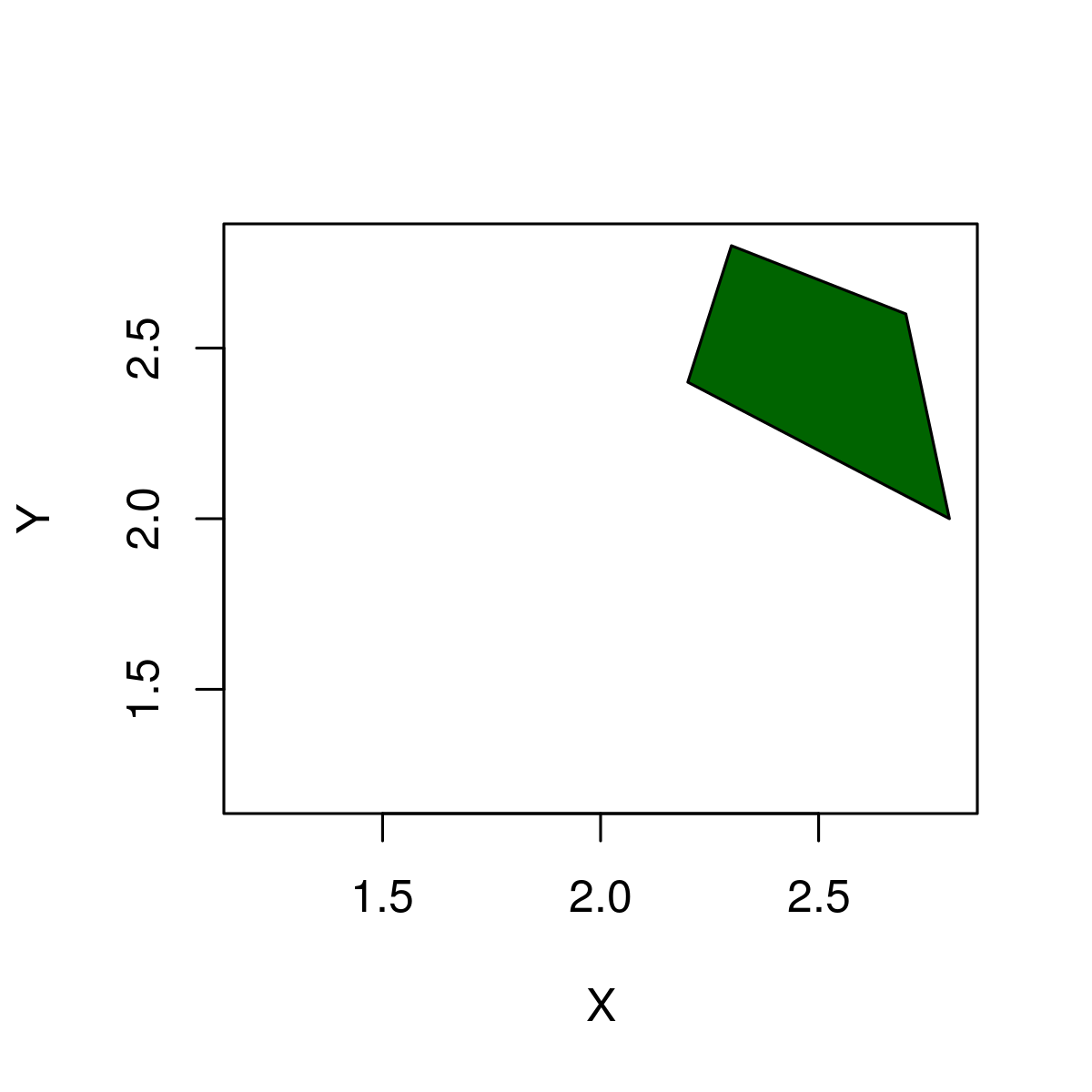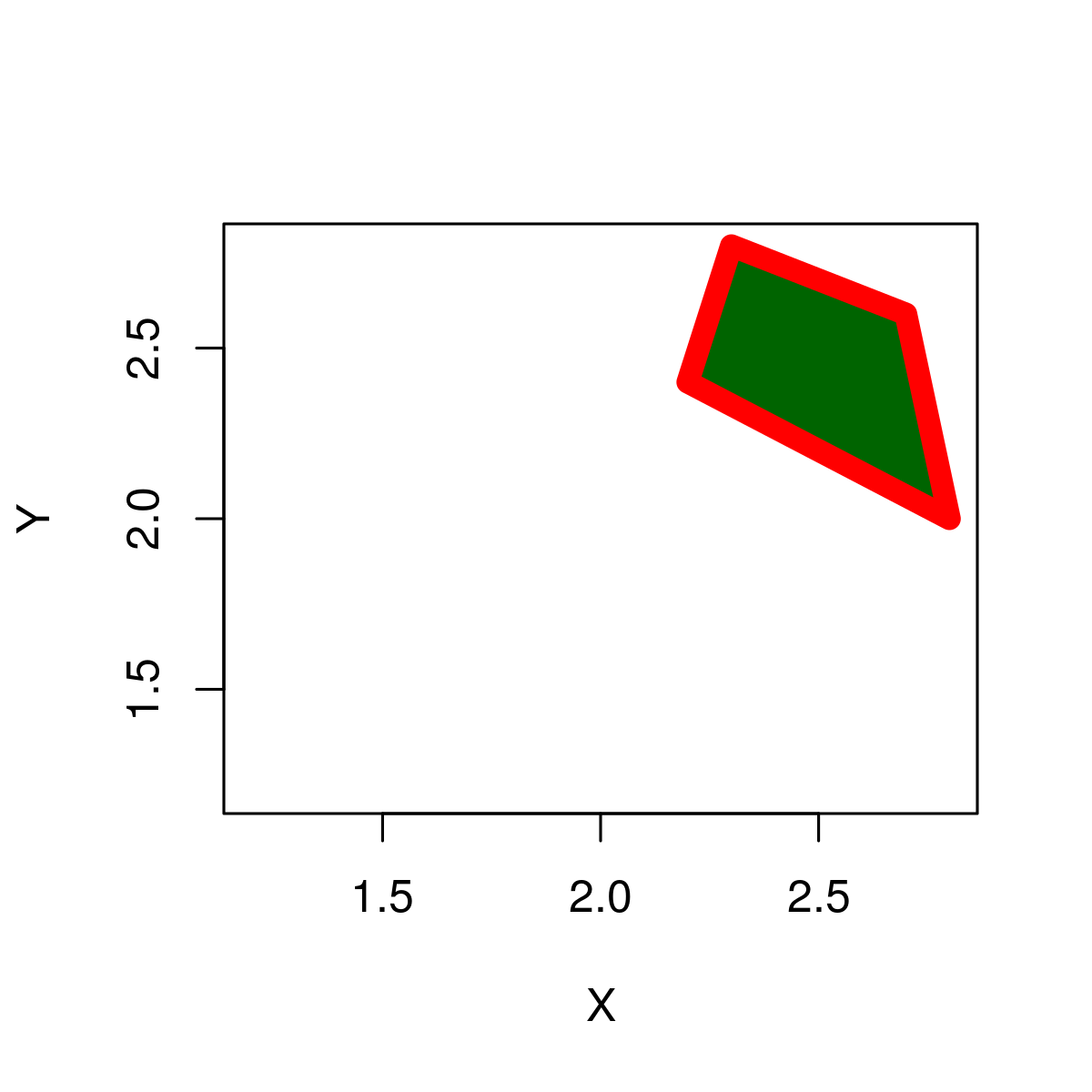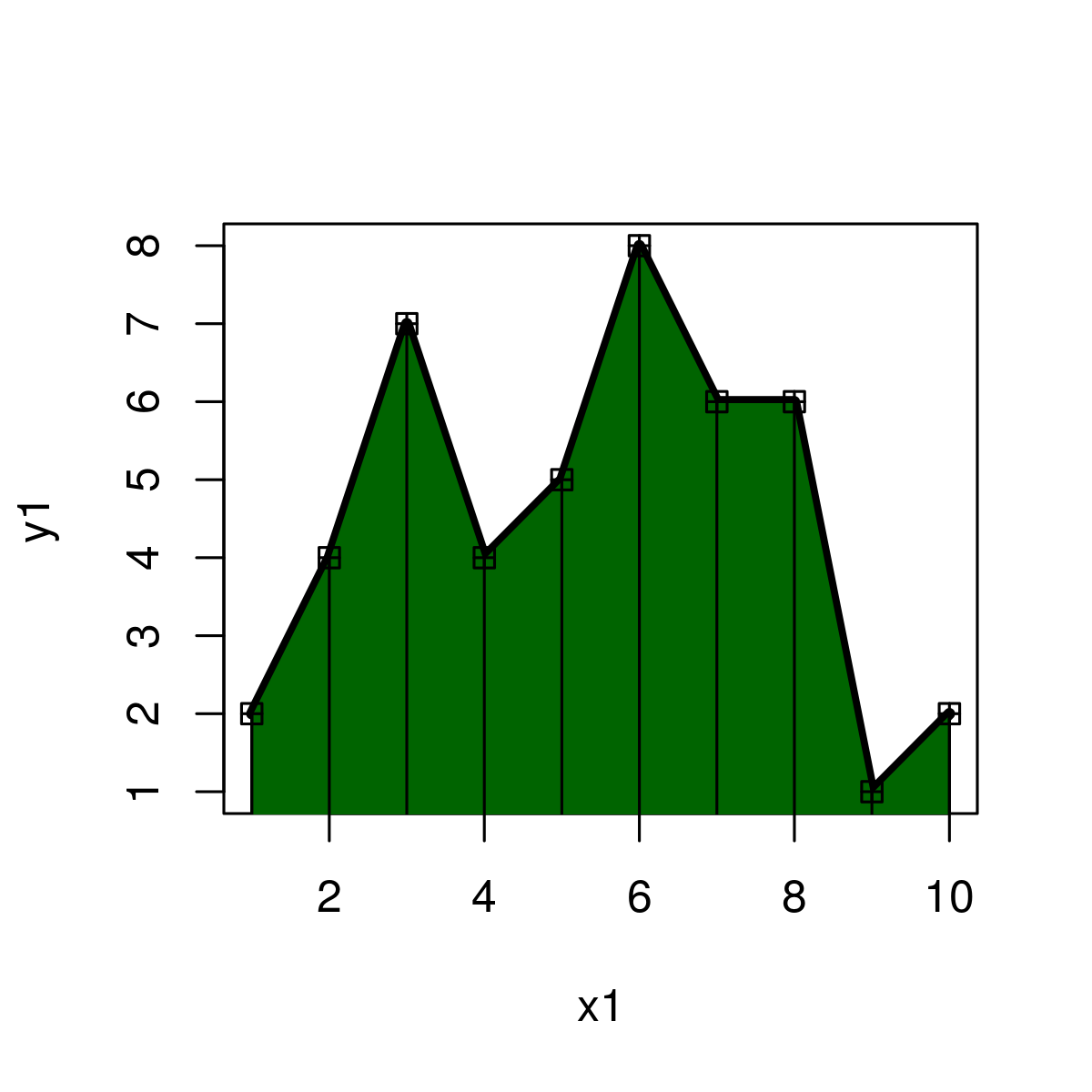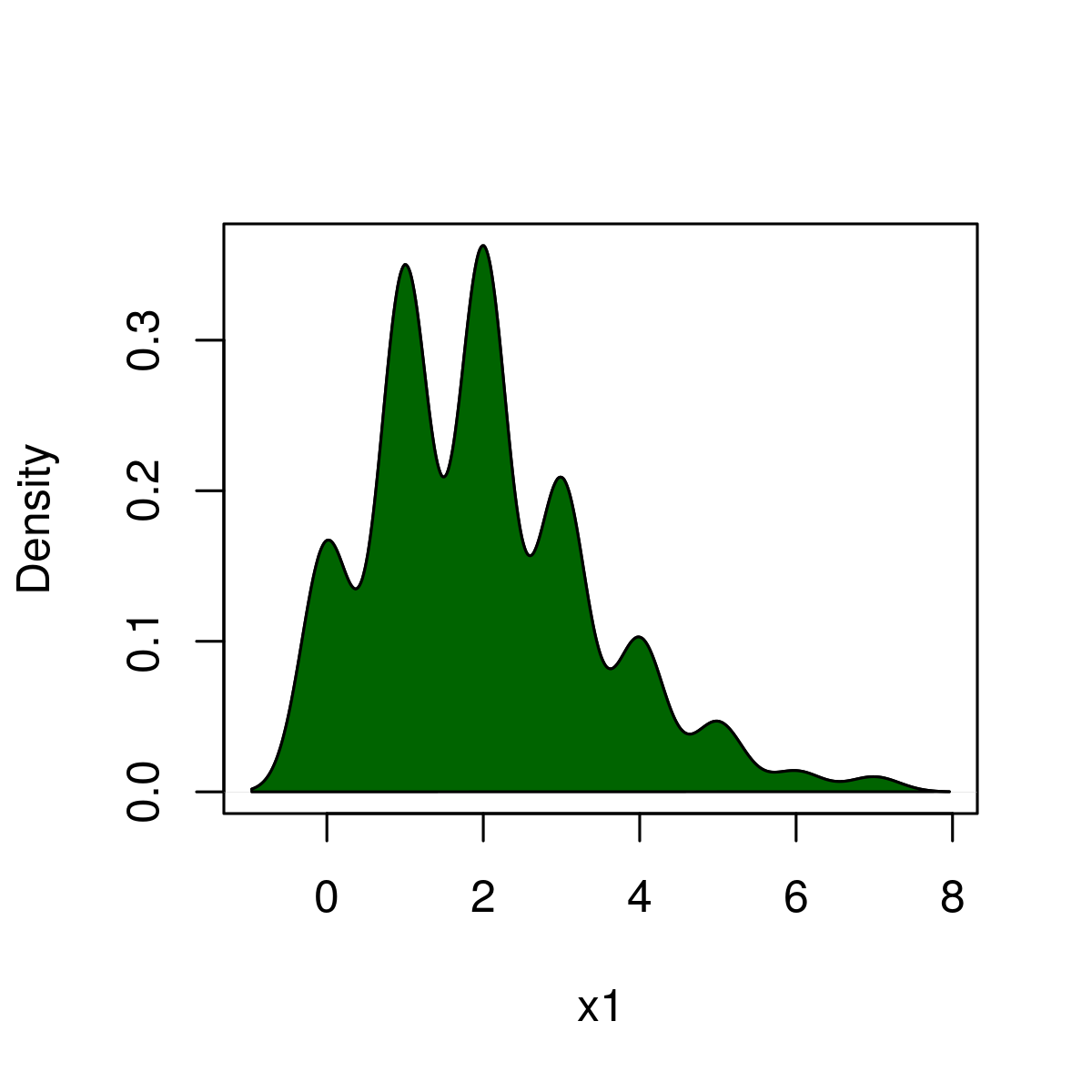# Draw a Polygon between specified points in R Programming – polygon() Function

`polygon()` function in R Language is used to plot a polygon between specified points in an existing plot.

Syntax: polygon(x_coordinates, y_coordinates)

Parameters :
x_coordinates, y_coordinates: x, y coordinates of plot to draw polygon

Returns: a polygon in a given plot

Example 1: Draw a Square Polygon in an R Plot

 `# R program to draw a polygon ` ` `  `# Draw an empty plot ` `plot``(2, 2, col = ``"white"``, xlab = ``"X"``, ylab = ``"Y"``)   ` ` `  `# Draw a polygon ` `polygon``(x = ``c``(2.7, 2.3, 2.2, 2.8),  ``# X-Coordinates of polygon  ` `        ``y = ``c``(2.6, 2.8, 2.4, 2),    ``# Y-Coordinates of polygon ` `        ``col = ``"darkgreen"``)  `

Output:Example 2: Color borders of polygon

 `# R program to draw a polygon ` ` `  `# Draw empty plot ` `plot``(2, 2, col = ``"white"``, xlab = ``"X"``, ylab = ``"Y"``)  ` ` `  `# Draw a polygon ` `polygon``(x = ``c``(2.7, 2.3, 2.2, 2.8),  ``# X-Coordinates of polygon ` `        ``y = ``c``(2.6, 2.8, 2.4, 2),    ``# Y-Coordinates of polygon ` `        ``col = ``"darkgreen"``,          ``# Color of polygon ` `        ``border = ``"red"``,             ``# Color of polygon border ` `        ``lwd = 8)                    ``# Thickness of border `

Output:Here, border specifies the border color and lwd specifies the border thickness.

Example 3: Draw frequency polygon

 `# R program to draw a polygon ` ` `  `# X values for frequency polygon ` `x1 <- 1:10                           ` ` `  `# Y values for frequency polygon ` `y1 <- ``c``(2, 4, 7, 4, 5, 8, 6, 6, 1, 2)   ` ` `  `# Plot frequency polygon ` `plot``(x1, y1,                                        ` `     ``type = ``"l"``,     ``# Set line type to line ` `     ``lwd = 4)         ``# Thickness of line ` `      `  `# X-Y-Coordinates of polygon ` `polygon``(``c``(1, x1, 10), ``c``(0, y1, 0),           ` `        ``col = ``"darkgreen"``)   ``# Color of polygon ` ` `  `# Add squares to frequency polygon ` `points``(x1, y1,                           ` `       ``cex = 1,    ``# Size of squares                              ` `       ``pch = 12)   ` `       ``segments``(x1, 0, x1, y1)   `

Output:Example 4: Draw polygon below density

 `# R program to draw a polygon ` ` `  `# Set seed for reproducibility ` `set.seed``(15000)       ` ` `  `# Sample size ` `N <- 1000              ` ` `  `# Draw random poisson distribution ` `x1 <- ``rpois``(N, 2)                            ` `plot``(``density``(x1),    ``# Draw density plot ` `     ``main = ``""``,      ``# No main title ` `     ``xlab = ``"x1"``)    ``# Set name of x-axis to x2 ` `      `  `# X-Coordinates of polygon ` `polygon``(``c``(``min``(``density``(x1)\$x), ``density``(x1)\$x),            ` `        ``c``(0, ``density``(x1)\$y),     ``# Y-Coordinates of polygon ` `        ``col = ``"darkgreen"``)       ``# Color of polygon `

Output:Here, above example is used to make Probability density function.

My Personal Notes arrow_drop_upCheck out this Author's contributed articles.

If you like GeeksforGeeks and would like to contribute, you can also write an article using contribute.geeksforgeeks.org or mail your article to contribute@geeksforgeeks.org. See your article appearing on the GeeksforGeeks main page and help other Geeks.

Please Improve this article if you find anything incorrect by clicking on the "Improve Article" button below.

Article Tags :

Be the First to upvote.

Please write to us at contribute@geeksforgeeks.org to report any issue with the above content.